# Mean State

Period Mean (original grids) [W/m2]
Model Period Mean (intersection) [W/m2]
Model Period Mean (complement) [W/m2]
Benchmark Period Mean (intersection) [W/m2]
Benchmark Period Mean (complement) [W/m2]
Bias [W/m2]
RMSE [W/m2]
Phase Shift [months]
Bias Score 
RMSE Score 
Seasonal Cycle Score 
Spatial Distribution Score 
Interannual Variability Score 
Overall Score 
Benchmark [-] 187.
CLM5PHSOFF [-] 181. 181. 0.00 186. 206. -4.89 15.2 0.808 0.72 0.50 0.90 0.94 0.80 0.73
CLM5PHSON [-] 182. 182. 0.00 186. 206. -4.45 15.1 0.823 0.73 0.49 0.90 0.94 0.80 0.73
Period Mean (original grids) [W/m2]
Model Period Mean (intersection) [W/m2]
Model Period Mean (complement) [W/m2]
Benchmark Period Mean (intersection) [W/m2]
Benchmark Period Mean (complement) [W/m2]
Bias [W/m2]
RMSE [W/m2]
Phase Shift [months]
Bias Score 
RMSE Score 
Seasonal Cycle Score 
Spatial Distribution Score 
Interannual Variability Score 
Overall Score 
Benchmark [-] 198.
CLM5PHSOFF [-] 194. 194. 0.00 198. 214. -3.94 14.6 0.751 0.70 0.51 0.91 0.97 0.85 0.74
CLM5PHSON [-] 195. 195. 0.00 198. 214. -2.80 14.2 0.767 0.71 0.52 0.91 0.96 0.85 0.74
Period Mean (original grids) [W/m2]
Model Period Mean (intersection) [W/m2]
Model Period Mean (complement) [W/m2]
Benchmark Period Mean (intersection) [W/m2]
Benchmark Period Mean (complement) [W/m2]
Bias [W/m2]
RMSE [W/m2]
Phase Shift [months]
Bias Score 
RMSE Score 
Seasonal Cycle Score 
Spatial Distribution Score 
Interannual Variability Score 
Overall Score 
Benchmark [-] 199.
CLM5PHSOFF [-] 195. 195. 0.00 198. 219. -3.15 15.5 0.706 0.80 0.57 0.90 0.99 0.75 0.76
CLM5PHSON [-] 196. 196. 0.00 198. 219. -2.87 15.6 0.787 0.80 0.57 0.89 1.0 0.75 0.76
Period Mean (original grids) [W/m2]
Model Period Mean (intersection) [W/m2]
Model Period Mean (complement) [W/m2]
Benchmark Period Mean (intersection) [W/m2]
Benchmark Period Mean (complement) [W/m2]
Bias [W/m2]
RMSE [W/m2]
Phase Shift [months]
Bias Score 
RMSE Score 
Seasonal Cycle Score 
Spatial Distribution Score 
Interannual Variability Score 
Overall Score 
Benchmark [-] 70.7
CLM5PHSOFF [-] 37.3 69.9 0.00 70.5 72.8 -0.883 18.9 0.310 0.91 0.77 0.98 1.0 0.74 0.86
CLM5PHSON [-] 73.1 73.3 0.00 70.5 72.8 1.86 18.4 0.331 0.91 0.77 0.98 1.0 0.74 0.86
Period Mean (original grids) [W/m2]
Model Period Mean (intersection) [W/m2]
Model Period Mean (complement) [W/m2]
Benchmark Period Mean (intersection) [W/m2]
Benchmark Period Mean (complement) [W/m2]
Bias [W/m2]
RMSE [W/m2]
Phase Shift [months]
Bias Score 
RMSE Score 
Seasonal Cycle Score 
Spatial Distribution Score 
Interannual Variability Score 
Overall Score 
Benchmark [-] 194.
CLM5PHSOFF [-] 179. 178. 0.00 190. 207. -11.6 19.3 1.25 0.53 0.44 0.80 0.96 0.77 0.66
CLM5PHSON [-] 175. 175. 0.00 190. 207. -11.2 19.1 1.25 0.54 0.44 0.80 0.96 0.77 0.66
Period Mean (original grids) [W/m2]
Model Period Mean (intersection) [W/m2]
Model Period Mean (complement) [W/m2]
Benchmark Period Mean (intersection) [W/m2]
Benchmark Period Mean (complement) [W/m2]
Bias [W/m2]
RMSE [W/m2]
Phase Shift [months]
Bias Score 
RMSE Score 
Seasonal Cycle Score 
Spatial Distribution Score 
Interannual Variability Score 
Overall Score 
Benchmark [-] 97.8
CLM5PHSOFF [-] 96.3 96.2 0.00 97.6 118. -1.32 17.7 0.230 0.92 0.79 0.98 0.99 0.71 0.86
CLM5PHSON [-] 100. 100. 0.00 97.6 118. 2.99 15.5 0.231 0.92 0.82 0.98 1.0 0.69 0.87
Period Mean (original grids) [W/m2]
Model Period Mean (intersection) [W/m2]
Model Period Mean (complement) [W/m2]
Benchmark Period Mean (intersection) [W/m2]
Benchmark Period Mean (complement) [W/m2]
Bias [W/m2]
RMSE [W/m2]
Phase Shift [months]
Bias Score 
RMSE Score 
Seasonal Cycle Score 
Spatial Distribution Score 
Interannual Variability Score 
Overall Score 
Benchmark [-] 139.
CLM5PHSOFF [-] 135. 135. 0.00 138. 149. -3.17 16.1 0.353 0.88 0.76 0.97 1.0 0.67 0.84
CLM5PHSON [-] 135. 135. 0.00 138. 149. -2.61 15.9 0.340 0.88 0.77 0.97 1.0 0.67 0.84
Period Mean (original grids) [W/m2]
Model Period Mean (intersection) [W/m2]
Model Period Mean (complement) [W/m2]
Benchmark Period Mean (intersection) [W/m2]
Benchmark Period Mean (complement) [W/m2]
Bias [W/m2]
RMSE [W/m2]
Phase Shift [months]
Bias Score 
RMSE Score 
Seasonal Cycle Score 
Spatial Distribution Score 
Interannual Variability Score 
Overall Score 
Benchmark [-] 181.
CLM5PHSOFF [-] 175. 175. 0.00 179. 205. -4.15 18.2 0.328 0.76 0.61 0.97 0.94 0.72 0.77
CLM5PHSON [-] 174. 174. 0.00 179. 205. -3.72 18.1 0.320 0.77 0.61 0.97 0.94 0.72 0.77
Period Mean (original grids) [W/m2]
Model Period Mean (intersection) [W/m2]
Model Period Mean (complement) [W/m2]
Benchmark Period Mean (intersection) [W/m2]
Benchmark Period Mean (complement) [W/m2]
Bias [W/m2]
RMSE [W/m2]
Phase Shift [months]
Bias Score 
RMSE Score 
Seasonal Cycle Score 
Spatial Distribution Score 
Interannual Variability Score 
Overall Score 
Benchmark [-] 62.8
CLM5PHSOFF [-] 62.7 63.1 0.00 63.2 54.4 -0.0956 15.9 0.179 0.93 0.79 0.99 0.99 0.74 0.87
CLM5PHSON [-] 64.7 65.0 0.00 63.2 54.4 0.684 15.6 0.199 0.93 0.79 0.99 0.99 0.75 0.87
Period Mean (original grids) [W/m2]
Model Period Mean (intersection) [W/m2]
Model Period Mean (complement) [W/m2]
Benchmark Period Mean (intersection) [W/m2]
Benchmark Period Mean (complement) [W/m2]
Bias [W/m2]
RMSE [W/m2]
Phase Shift [months]
Bias Score 
RMSE Score 
Seasonal Cycle Score 
Spatial Distribution Score 
Interannual Variability Score 
Overall Score 
Benchmark [-] 198.
CLM5PHSOFF [-] 190. 190. 0.00 198. 197. -8.29 16.0 0.373 0.83 0.76 0.97 1.0 0.71 0.84
CLM5PHSON [-] 192. 192. 0.00 198. 197. -7.59 15.6 0.385 0.85 0.76 0.97 1.0 0.72 0.84
Period Mean (original grids) [W/m2]
Model Period Mean (intersection) [W/m2]
Model Period Mean (complement) [W/m2]
Benchmark Period Mean (intersection) [W/m2]
Benchmark Period Mean (complement) [W/m2]
Bias [W/m2]
RMSE [W/m2]
Phase Shift [months]
Bias Score 
RMSE Score 
Seasonal Cycle Score 
Spatial Distribution Score 
Interannual Variability Score 
Overall Score 
Benchmark [-] 120.
CLM5PHSOFF [-] 115. 115. 0.00 118. 145. -2.77 13.7 0.238 0.92 0.84 0.98 0.99 0.66 0.87
CLM5PHSON [-] 113. 113. 0.00 118. 145. -2.30 13.6 0.227 0.92 0.84 0.98 0.99 0.66 0.87
Period Mean (original grids) [W/m2]
Model Period Mean (intersection) [W/m2]
Model Period Mean (complement) [W/m2]
Benchmark Period Mean (intersection) [W/m2]
Benchmark Period Mean (complement) [W/m2]
Bias [W/m2]
RMSE [W/m2]
Phase Shift [months]
Bias Score 
RMSE Score 
Seasonal Cycle Score 
Spatial Distribution Score 
Interannual Variability Score 
Overall Score 
Benchmark [-] 163.
CLM5PHSOFF [-] 147. 136. 0.00 142. 173. -5.43 16.4 0.384 0.81 0.70 0.96 1.0 0.72 0.81
CLM5PHSON [-] 137. 137. 0.00 142. 173. -4.59 16.0 0.394 0.82 0.70 0.96 1.0 0.71 0.82
Period Mean (original grids) [W/m2]
Model Period Mean (intersection) [W/m2]
Model Period Mean (complement) [W/m2]
Benchmark Period Mean (intersection) [W/m2]
Benchmark Period Mean (complement) [W/m2]
Bias [W/m2]
RMSE [W/m2]
Phase Shift [months]
Bias Score 
RMSE Score 
Seasonal Cycle Score 
Spatial Distribution Score 
Interannual Variability Score 
Overall Score 
Benchmark [-] 177.
CLM5PHSOFF [-] 163. 163. 0.00 175. 217. -12.2 17.9 0.296 0.71 0.76 0.98 0.96 0.68 0.81
CLM5PHSON [-] 162. 162. 0.00 175. 217. -12.0 18.0 0.296 0.71 0.76 0.98 0.96 0.67 0.81
Period Mean (original grids) [W/m2]
Model Period Mean (intersection) [W/m2]
Model Period Mean (complement) [W/m2]
Benchmark Period Mean (intersection) [W/m2]
Benchmark Period Mean (complement) [W/m2]
Bias [W/m2]
RMSE [W/m2]
Phase Shift [months]
Bias Score 
RMSE Score 
Seasonal Cycle Score 
Spatial Distribution Score 
Interannual Variability Score 
Overall Score 
Benchmark [-] 69.7
CLM5PHSOFF [-] 68.1 67.9 0.00 69.6 71.1 -1.70 17.5 0.0891 0.92 0.78 0.99 0.99 0.75 0.87
CLM5PHSON [-] 70.7 70.6 0.00 69.6 71.1 -0.616 17.1 0.0938 0.92 0.78 0.99 0.98 0.75 0.87
Period Mean (original grids) [W/m2]
Model Period Mean (intersection) [W/m2]
Model Period Mean (complement) [W/m2]
Benchmark Period Mean (intersection) [W/m2]
Benchmark Period Mean (complement) [W/m2]
Bias [W/m2]
RMSE [W/m2]
Phase Shift [months]
Bias Score 
RMSE Score 
Seasonal Cycle Score 
Spatial Distribution Score 
Interannual Variability Score 
Overall Score 
Benchmark [-] 204.
CLM5PHSOFF [-] 193. 193. 0.00 201. 219. -7.37 17.0 0.480 0.76 0.68 0.94 0.99 0.75 0.80
CLM5PHSON [-] 192. 192. 0.00 201. 219. -6.91 16.8 0.470 0.77 0.68 0.94 0.99 0.75 0.80
Period Mean (original grids) [W/m2]
Model Period Mean (intersection) [W/m2]
Model Period Mean (complement) [W/m2]
Benchmark Period Mean (intersection) [W/m2]
Benchmark Period Mean (complement) [W/m2]
Bias [W/m2]
RMSE [W/m2]
Phase Shift [months]
Bias Score 
RMSE Score 
Seasonal Cycle Score 
Spatial Distribution Score 
Interannual Variability Score 
Overall Score 
Benchmark [-] 150.
CLM5PHSOFF [-] 145. 145. 0.00 148. 192. -3.12 14.2 0.274 0.93 0.79 0.98 0.99 0.71 0.87
CLM5PHSON [-] 145. 145. 0.00 148. 192. -2.06 13.6 0.274 0.94 0.80 0.98 0.99 0.70 0.87
Period Mean (original grids) [W/m2]
Model Period Mean (intersection) [W/m2]
Model Period Mean (complement) [W/m2]
Benchmark Period Mean (intersection) [W/m2]
Benchmark Period Mean (complement) [W/m2]
Bias [W/m2]
RMSE [W/m2]
Phase Shift [months]
Bias Score 
RMSE Score 
Seasonal Cycle Score 
Spatial Distribution Score 
Interannual Variability Score 
Overall Score 
Benchmark [-] 110.
CLM5PHSOFF [-] 108. 108. 0.00 110. 117. -1.86 15.9 0.110 0.91 0.82 0.99 1.0 0.71 0.87
CLM5PHSON [-] 111. 111. 0.00 110. 117. 1.63 13.7 0.114 0.93 0.84 0.99 1.0 0.68 0.88
Period Mean (original grids) [W/m2]
Model Period Mean (intersection) [W/m2]
Model Period Mean (complement) [W/m2]
Benchmark Period Mean (intersection) [W/m2]
Benchmark Period Mean (complement) [W/m2]
Bias [W/m2]
RMSE [W/m2]
Phase Shift [months]
Bias Score 
RMSE Score 
Seasonal Cycle Score 
Spatial Distribution Score 
Interannual Variability Score 
Overall Score 
Benchmark [-] 161.
CLM5PHSOFF [-] 158. 158. 0.00 161. 163. -3.08 14.7 0.214 0.93 0.82 0.99 0.99 0.72 0.88
CLM5PHSON [-] 159. 159. 0.00 161. 163. -1.43 14.3 0.202 0.93 0.82 0.99 0.98 0.70 0.87
Period Mean (original grids) [W/m2]
Model Period Mean (intersection) [W/m2]
Model Period Mean (complement) [W/m2]
Benchmark Period Mean (intersection) [W/m2]
Benchmark Period Mean (complement) [W/m2]
Bias [W/m2]
RMSE [W/m2]
Phase Shift [months]
Bias Score 
RMSE Score 
Seasonal Cycle Score 
Spatial Distribution Score 
Interannual Variability Score 
Overall Score 
Benchmark [-] 179.
CLM5PHSOFF [-] 174. 174. 0.00 178. 218. -4.08 14.5 0.772 0.72 0.45 0.90 0.96 0.80 0.71
CLM5PHSON [-] 173. 173. 0.00 178. 218. -3.71 14.5 0.798 0.73 0.45 0.90 0.96 0.80 0.71
Period Mean (original grids) [W/m2]
Model Period Mean (intersection) [W/m2]
Model Period Mean (complement) [W/m2]
Benchmark Period Mean (intersection) [W/m2]
Benchmark Period Mean (complement) [W/m2]
Bias [W/m2]
RMSE [W/m2]
Phase Shift [months]
Bias Score 
RMSE Score 
Seasonal Cycle Score 
Spatial Distribution Score 
Interannual Variability Score 
Overall Score 
Benchmark [-] 185.
CLM5PHSOFF [-] 179. 179. 0.00 184. 202. -5.01 16.0 0.635 0.80 0.64 0.92 0.97 0.71 0.78
CLM5PHSON [-] 180. 180. 0.00 184. 202. -4.33 15.8 0.702 0.82 0.64 0.91 0.97 0.72 0.78
Period Mean (original grids) [W/m2]
Model Period Mean (intersection) [W/m2]
Model Period Mean (complement) [W/m2]
Benchmark Period Mean (intersection) [W/m2]
Benchmark Period Mean (complement) [W/m2]
Bias [W/m2]
RMSE [W/m2]
Phase Shift [months]
Bias Score 
RMSE Score 
Seasonal Cycle Score 
Spatial Distribution Score 
Interannual Variability Score 
Overall Score 
Benchmark [-] 178.
CLM5PHSOFF [-] 173. 172. 0.00 179. 169. -7.64 20.8 0.405 0.79 0.71 0.96 1.0 0.69 0.81
CLM5PHSON [-] 176. 175. 0.00 179. 169. -8.13 21.0 0.401 0.78 0.71 0.96 1.0 0.69 0.81

# Temporally integrated period mean

BENCHMARK MEAN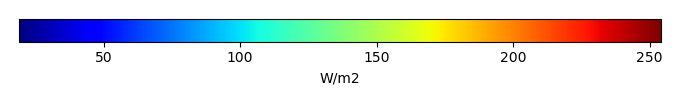MODEL MEANBIAS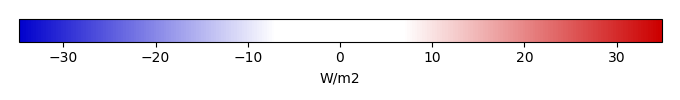BIAS SCORERMSE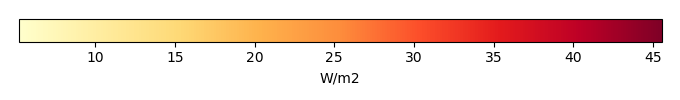RMSE SCOREBENCHMARK INTERANNUAL VARIABILITY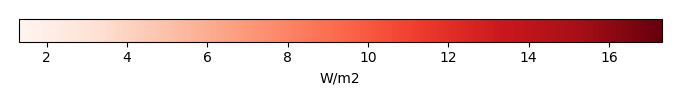MODEL INTERANNUAL VARIABILITYINTERANNUAL VARIABILITY SCOREBENCHMARK MAX MONTHMODEL MAX MONTHDIFFERENCE IN MAX MONTH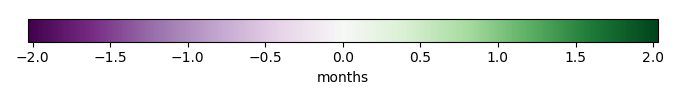SEASONAL CYCLE SCORESPATIAL TAYLOR DIAGRAMMODEL COLORS# Spatially integrated regional mean

MODEL COLORSREGIONAL MEANANNUAL CYCLEMONTHLY ANOMALYANNUAL CYCLE# All Models

BenchmarkCLM5PHSOFFCLM5PHSON# Data Information

creation_date: Thu May 8 23:03:44 PDT 2014

source_file: This product is generated from monthly 1 degree CERES EBAF Radiation observations